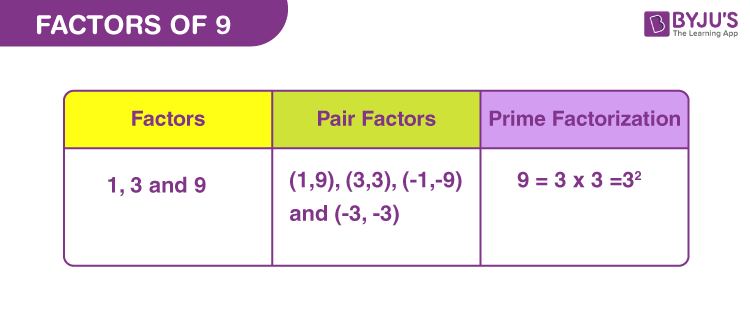# Factors of 9

Factors of 9 are those real numbers that can divide the original number evenly. Since we know that 9 is a composite number, therefore it will have factors of more than two. Also, 9 is the perfect square such that 9 = 3 x 3 = 32. Thus, we can conclude that 3 is a factor of 9.

9 divided by 3 is 3 ⇒ 9 ÷ 3 = 3

Similarly, we will come across the pair factors of 9 in this article. Pair factors are those sets of numbers that on multiplication result in the original number. Prime factors are the prime numbers that divide the original number. Let us learn at BYJU’S, how to find the factors and pair factors along with prime factorisation, easily.## How to Find Factors of 9?

Factors of any number are those natural numbers that can divide the original number without leaving any remainder. Since, there are only 9 natural numbers, from 1 to 9, thus we can check the factors easily and quickly here.

We need to divide 9 by each of the counting numbers and check if the resultant quotient is a whole number or a fraction. If the quotient is a fraction, then the divisor is not a factor.

9 ÷ 1 = 9 [Whole number]

9 ÷ 2 = 4.5 [Fraction]

9 ÷ 3 = 3 [Whole number]

9 ÷ 4 = 2.25 [Fraction]

9 ÷ 5 = 1.8 [Fraction]

9 ÷ 6 = 1.5 [Fraction]

9 ÷ 7 = 1.285.. [Fraction]

9 ÷ 8 = 1.125 [Fraction]

9 ÷ 9 = 1 [Whole number]

Thus, we can conclude that only 1, 3 and 9 are the factors of 9.

## Pair Factors of 9

To find the pair factors, multiply the two numbers in a pair to get the original number as 9.

1 × 9 = 9

3 × 3 = 9

Therefore, the pair factors are (1, 9) and (3, 3). These are the positive pair factors.

Likewise, we can also consider negative pair factors since two negative factors will result in a positive value after multiplication.

-1 × -9 = 9

-3 × -3 = 9

Therefore, the negative pair factors are (-1, -9) and (-3, -3).

## Prime Factorisation of 9

To find the prime factors of 9 we need to divide it by each of the prime numbers till the remainder is 1.

Step 1: Divide 9 by the smallest prime factor. As we know, the smallest prime number is 2 but if we divide 9 by 2, we will get a fraction. Thus, here we will take the next smallest prime factor, i.e. 3.

9/3 = 3

Step 2: Again, divide 3 by the smallest prime factor, i.e., 3.

3/3 = 1

Step 3: We cannot divide 1 by any prime factor, further. Therefore,

 Prime factorisation of 9 = 3 x 3 = 32

## Video Lesson on Prime Factors## Solved Examples

Q.1: If there are 9 mangoes to be distributed among 3 children. How many mangoes does each child get?

Solution: Given,

Number of mangoes = 9

Number of children = 3

Each child will get = 9/3 = 3 mangoes

Q.2: What is the sum of all the factors of 9?

Solution: The factors of nine are 1, 3 and 9.

Sum = 1 + 3 + 9 = 13

Therefore, 13 is the required sum.

Q.3: What are the common factors of 9 and 27?

Answer: To find the common factors, we need to write down the factors of each of the given numbers.

9 → 1, 3, and 9

27 → 1, 3, 9, and 27

Hence, we can see all the factors of 9 are the common factors of 27.

## Frequently Asked Questions on Factors of 9

Q1

### What are the total factors of 9?

The factors of 9 are 1, 3 and 9.

Q2

### Are multiples and factors of 9 the same?

Multiples of 9 are the values that are divisible by the original number, whereas factors divide the original number evenly. Therefore, both are not the same.

Q3

### What are the multiples of 9?

The first 10 multiples of 9 are 9, 18, 27, 36, 45, 54, 63, 72, 81 and 90.

Q4

### What are the prime factors of 9?

The prime factorisation of 9 is 3 x 3 or 32.

Q5

### What is the average of factors of 9?

Average is the sum of all the values divided by a number of values.
Average of factor of 9 = (1+3+9)/3 = 13/3

Test your Knowledge on Factors of 9